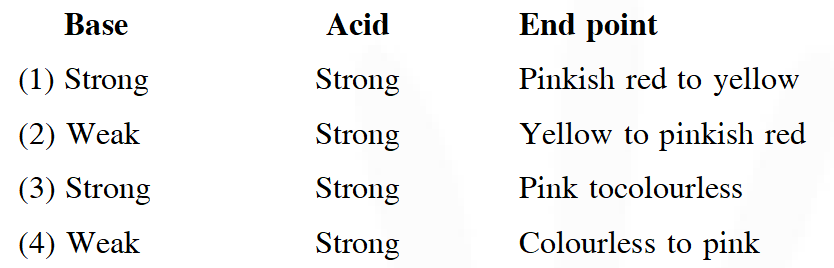# Redox Reaction - JEE Main Previous Year Questions with Solutions

JEE Main Previous Year Papers Questions of Chemistry With Solutions are available at eSaral.   Simulator   Previous Years AIEEE/JEE Mains Questions
Q. Consider the following reaction: $\mathrm{xMnO}_{4}^{-}+\mathrm{yC}_{2} \mathrm{O}_{4}^{2-}+\mathrm{ZH}^{+} \rightarrow$ $\mathrm{xMn}^{2+}+2 \mathrm{yCO}_{2}+\frac{\mathrm{Z}}{2} \mathrm{H}_{2} \mathrm{O}$ The values of x, y and z in the reaction are respectively :- (1) 5,2 and 16 (2) 2,5 and 8 (3) 2, 5 and 16 (4) 5,2 and 8 [JEE(Main)-2013]
Ans. (3) Fact
Q. Given : $\mathrm{X} \mathrm{Na}_{2} \mathrm{HAsO}_{3}+\mathrm{Y} \mathrm{NaBrO}_{3}+\mathrm{ZHCl} \rightarrow \mathrm{NaBr}$ $+\mathrm{H}_{3} \mathrm{AsO}_{4}+\mathrm{NaCl}$ The values of X, Y and Z in the above redox reaction are respectively : (1) 2, 1, 3 (2) 3, 1, 6 (3) 2, 1, 2 (4) 3, 1, 4 [JEE(Main-online)-2013]
Ans. (2) Fact
Q. In which of the following reaction $\mathrm{H}_{2} \mathrm{O}_{2}$ acts as a reducing agent ? (1) $\mathrm{H}_{2} \mathrm{O}_{2}+2 \mathrm{H}^{+}+2 \mathrm{e}^{-} \rightarrow 2 \mathrm{H}_{2} \mathrm{O}$ (2) $\mathrm{H}_{2} \mathrm{O}_{2}-2 \mathrm{e}^{-} \rightarrow \mathrm{O}_{2}+2 \mathrm{H}^{+}$ (3) $\mathrm{H}_{2} \mathrm{O}_{2}+2 \mathrm{e}^{-} \rightarrow 2 \mathrm{OH}^{-}$ (4) $\mathrm{H}_{2} \mathrm{O}_{2}+2 \mathrm{OH}^{-}-2 \mathrm{e}^{-} \rightarrow \mathrm{O}_{2}+2 \mathrm{H}_{2} \mathrm{O}$ (1) (1), (3) (2) (2), (4) (3) (1), (2) (4) (3), (4) [JEE(Main-online)-2014]
Ans. (2) Fact
Q. Consider the reaction $\mathrm{H}_{2} \mathrm{SO}_{3(\mathrm{aq})}+\mathrm{Sn}_{(\mathrm{aq})}^{4+}+\mathrm{H}_{2} \mathrm{O}_{(\mathrm{l})} \rightarrow \mathrm{Sn}_{(\mathrm{aq})}^{2+}+\mathrm{HSO}_{4(\mathrm{aq})}^{-}+3 \mathrm{H}_{(\mathrm{aq})}^{+}$ Which of the following statements is correct? (1) $\mathrm{H}_{2} \mathrm{SO}_{3}$ is the reducing agent because it undergoes oxidation (2) $\mathrm{H}_{2} \mathrm{SO}_{3}$ is the reducing agent because it undergoes reduction (3) $\mathrm{Sn}^{4+}$ is the reducing agent because it undergoes oxidation (4) $\mathrm{Sn}^{4+}$ is the oxidizing agent because it undergoes oxidation [JEE(Main-online)-2014]
Ans. (1) Fact
Q. How many electrons are involved in the following redox reaction ? $\mathrm{Cr}_{2} \mathrm{O}_{7}^{2-}+\mathrm{Fe}^{2+}+\mathrm{C}_{2} \mathrm{O}_{4}^{2-} \rightarrow \mathrm{Cr}^{3+}+\mathrm{Fe}^{3+}+\mathrm{CO}_{2}$ (Unbalanced) (1) 3 (2) 4 (3) 5 (4) 6 [JEE(Main-online)-2014]
Ans. (4) Fact
Q. Which of the following reactions is an example of a redox reaction ? (1) $\mathrm{XeF}_{4}+\mathrm{O}_{2} \mathrm{F}_{2} \rightarrow \mathrm{XeF}_{6}+\mathrm{O}_{2}$ (2) $\mathrm{XeF}_{2}+\mathrm{PF}_{5} \rightarrow[\mathrm{XeF}]^{+} \mathrm{PF}_{6}^{-}$ (3) $\mathrm{XeF}_{6}+\mathrm{H}_{2} \mathrm{O} \rightarrow \mathrm{XeOF}_{4}+2 \mathrm{HF}$ (4) $\mathrm{XeF}_{6}+2 \mathrm{H}_{2} \mathrm{O} \rightarrow \mathrm{XeO}_{2} \mathrm{F}_{2}+4 \mathrm{HF}$ [JEE-Main 2017]
Ans. (1) In the reaction $\stackrel{+4}{\mathrm{X}} \mathrm{e} \mathrm{F}_{4}+\mathrm{O}_{2} \mathrm{F}_{2} \rightarrow \stackrel{+6}{\mathrm{X}} \mathrm{eF}_{6}+\mathrm{O}_{2}^{0}$ Xenon undergoes oxidation while oxygen undergoes reduction.
Q. An alkali is titrated against an acid with methyl orange as indicator, which of the following is a correct combination ?[JEE-Main 2018]
Ans. (2) Methyl orange shows Red(pinkish) color in Acidic medium & yellow color in basic medium since original solution is basic so initial color  yellow & Titrated with acid so Final color  pinkish (red)

baba ramdev
March 25, 2022, 4:28 p.m.
teri maa fact bsdk
HEW
Feb. 10, 2022, 7:22 p.m.
Hi my name is Abhishek Kumar and I studied in class 11th I have many doubts about the Chemistry jegdyuuew kdshfygqw jg uaYNHGDJ H
👨
Jan. 22, 2021, 8:32 a.m.
SAILESH
Sept. 30, 2020, 8:55 p.m.
Thank u very much please increase the content of the questions so that it will be very helpful
Pranav
Sept. 10, 2020, 10:54 a.m.
Answer of question are given in detailed explanation.
Dhruvi
July 11, 2020, 12:12 p.m.
Good question Which help me a lot thanks
Jai jaiswal
June 6, 2020, 8:42 a.m.
Worst people,not giving questions. Damn,waste
Jay jaiswal
April 26, 2020, 2:50 p.m.
Very very thankful for the question dear sir/ma'am Can u give me some more q. Please